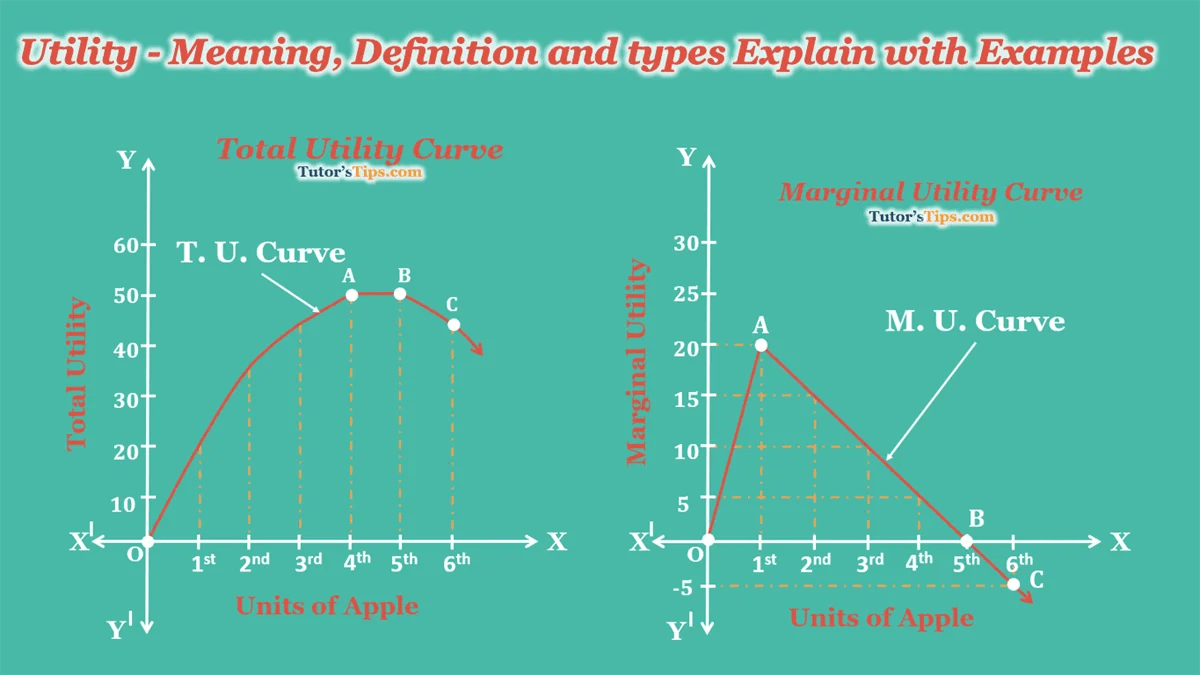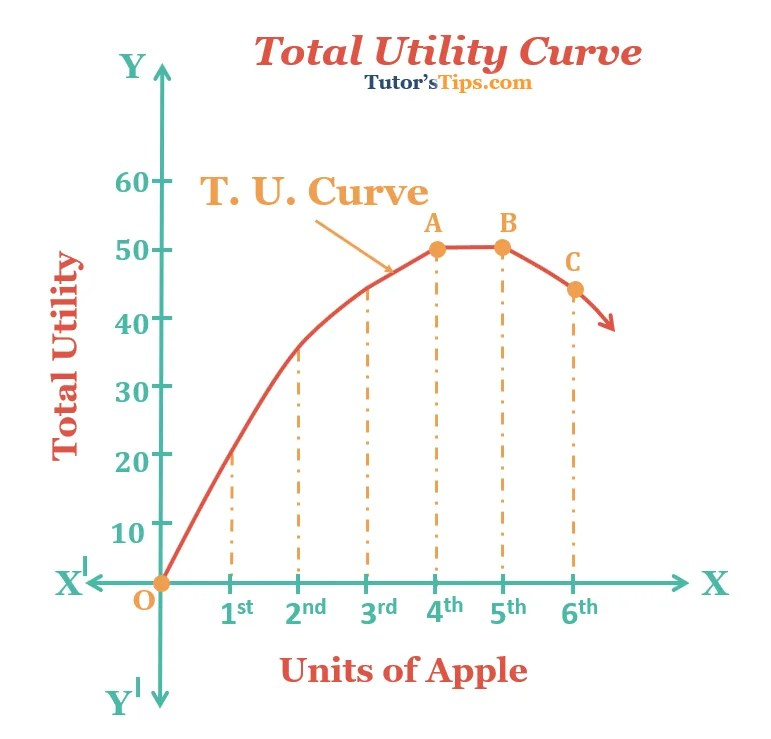# Utility – Meaning, Definition and its typesThe term Utility in economics refers to the satisfaction derived by a person from the consumption of any good or service at a given price and given time. It varies from person to person, place to place and time to time.

In other words, when a commodity is capable of satisfying a want, it can be said as it has a utility. It is measured in cardinal (numerical e.g 1,2,3,4,5 etc.) terms.

## Definition: –

According to Prof. Hobson, “Utility is the ability of a good to satisfy a want.”

It is impossible to measure the satisfaction of a person as it varies from person to person. Still, this concept is very useful in understanding consumer behaviour.

## Types of Utility:

It can be divided into two types. these are explained as follows: –

### 1. Total utility:

It refers to the total satisfaction obtained from the consumption of all possible units of a commodity. For example, if consumption of one apple gives a person satisfaction of 10 units and consumption of another apple gives the satisfaction of 8 units, the total satisfaction from two apples will be (10+8) = 18 units. If one more apple gives him the satisfaction of 6 units, then total satisfaction becomes (10+8+6)=24 units.

TUn = U1+U2+U3+………..+Un

Where,

TUn  = Total utility from n units of a given commodity

n = number of units consumed

U1, U2, U3 ………….Un = utility from 1st, 2nd, 3rd to an nth unit of commodity.

### 2. Marginal utility:

It refers to the additional utility derived from the consumption of one more unit of a given commodity in a given time period.

MU can be calculated as

MUn = TUn – TUn-1

Where MUn = marginal utility from an nth unit

TUn = Total utility from n units

TUn-1 = Total utility from n-1 units

In simple words, MU is the change in total utility when more unit of a given commodity is consumed.

Therefore,

“MU= Change in TU/ Change in units consumed”

## Illustration of Total and Marginal Utility:

 Units of Apples T. U. M. U. Remarks 0 0 —— 1st 20 20 (20-0) Positive + 2nd 35 15 (35-20) 3rd 45 10 (45-35) 4th 50 5 (50-45) 5th 50 0 (50-50) Zero 0 6th 45 -5 (45-50) Negative –

### Explanation:

In the above table, the consumer consumes the 1st apple and it gives him the satisfaction of 20 units and consumption of 2nd apple gives the satisfaction of 15 units. So, the total satisfaction gets from the consumption of two apples will be (20+15) = 35 units. When he ate a 3rd apple it gives him the satisfaction of 10 units, then total satisfaction gets from all units of apple becomes (20+15+10) = 45 units.

After that, When he ate the 4th unit of apple it gives him the satisfaction of 5 units, then total satisfaction gets from all units of apple becomes (20+15+10+5) = 50 units. After that when he ate the 5th unit of apple it gives him the satisfaction of 0 units, then total satisfaction gets from all units of apple becomes (20+15+10+5+0) = 50 units. and after that when he ate the 6th unit of apple it gives him the satisfaction of -5 units, then total satisfaction gets from all units of apple becomes (20+15+10+5+0+(-5)) = 45 units.

But the Marginal Utility has there different types of situation: –

1. Positive
2. Zero
3. Negative

#### These all explain further as following with the help of the above table: –

From the consumption of 1st unit to the 4th unit of the commodity, the M.U. driven from the consumption of these units is positive and the T.U. is increasing in the diminishing rate. when the consumer consumes the 5th unit of the commodity, the M.U. become zero and T.U. has no change. After that when consumer consumes the 6th unit of the commodity, the M.U. become Negative and T.U. is decreasing.

### Total Utility Curve: –In the above graph, we take units of apple on X-axis and T.U. on the Y-axis. The graph shows that from the point “O” to the point “A” the T.U. is increasing in the diminishing rate and from the point, “A” to the point “B” the T.U. has no change. after that from the point, “B” to the point “C” the  T.U. is decreasing.

### Marginal Utility Curve: –In the above graph, we take units of apple on X-axis and M.U. on the Y-axis. The graph shows that from the point “O” to the point “A” the M.U. driven the positive satisfaction and from the point, “A” to the point “B” the M.U. become zero. After that from the point, “B” to the point “C” the M.U. become Negative.